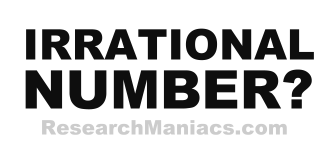Is 91 an Irrational Number?The number 91 is an irrational number if 91 canNOT be expressed as a ratio, as in irRATIOnal.

A quotient is the result you get when you divide one number by another number. For 91 to be an irrational number, the quotient of two integers canNOT equal 91.

In other words, for 91 to be an irrational number, 91 canNOT be expressed as a ratio where both the numerator and denominator are integers (whole numbers).

The number 91 can be expressed as 91/1 where 91 is the numerator and 1 is denominator.

Thus, the answer to the question "Is 91 an irrational number?" is NO.

Irrational Number?
Enter another number to see if it is irrational:

Is 92 an Irrational Number?
See information about the next number on our list.